# [worth collecting] for Python beginners, sort out the common errors of beginners + Python Mini applet! (code attached)

2022-01-31 09:51:56

## Introduction

I don't know what the best programming language in the world is ？

But life is short , I use Python！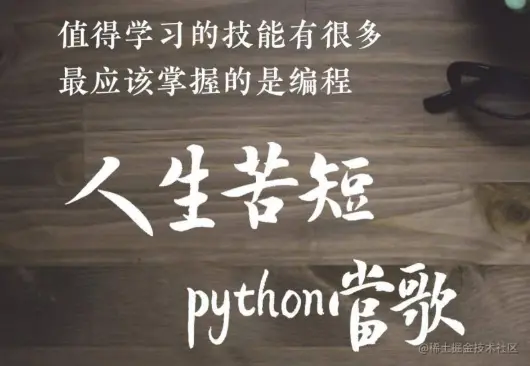** Write code , Mistakes are inevitable ,** The key is how to quickly locate errors , solve bug. Error message , Sometimes it doesn't provide effective information , Especially new programming

hand , Often make some low-level mistakes , such as Incorrect indentation , Missing quotation marks , Incomplete parentheses etc. , Here are some code mistakes that novices often make , I hope you're just getting started

My classmates have a little help ~ In addition to summarizing the common mistakes of novice programming, some simple novice small projects will be attached for everyone to learn ！

## Text

One 、 Novice programming error

1） Basic mistakes that novices often make

1. Missing colon ：

``````a=[1,2,3,4,5,6,7,8,9]
for i in range(0,len(a))
for j in range(i+1,len(a)):
if a[j]<a[i]:
a[i],a[j]=a[j],a[i]
print(a)
Copy code ``````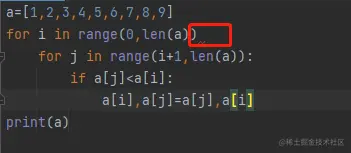Error message ：# Grammar mistakes ： invalid syntax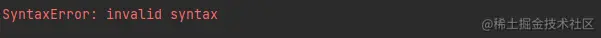### 2. Incorrect indentation

For class definitions 、 Function definition 、 Flow control statement 、 Exception handling statements, etc , The colon at the end of the line and the indent of the next line , Indicates the beginning of the next block of code , and

The end of the indentation indicates the end of the code block . Code with the same indentation is considered a block of code .

``````num = 2
if num < 5:
print(num)
Copy code ``````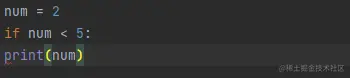Error message ：# The indentation error ： Blocks that need to be indented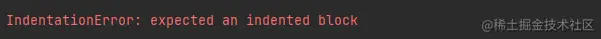### 3. The symbol is Chinese

Like a colon 、 Brackets are Chinese symbols, etc .

``````for i in range(2,102)：
print(i)
Copy code ``````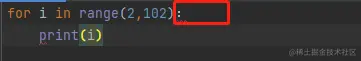​ Error message ：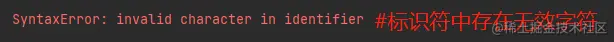### 4. Wrong data type

Common examples are : Splicing different types of data, etc .

``````name = 'xiaoming'
age = 16
print(name+age)
Copy code ``````​ Error message ：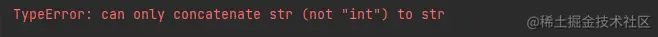### 5. Misspelling variable or function name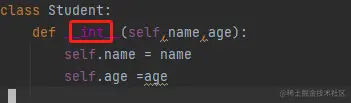### 6. Use keywords as file names 、 Class name 、 Function name or variable name .

Class name 、 Function name or variable name , Out of commission Python Language keywords . file name , Cannot conflict with standard library .

Python3 The key words are ：

``````and, as, assert, break, class, continue, def, del, elif,else, except, False, finally, for, from, global, if, import, in, is, lambda,None, nonlocal, not, or, pass, raise, return, True, try, while, with, yield
Copy code ``````

error ：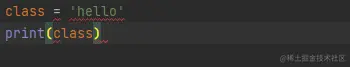### 7."=" treat as “==” Use

"=" Is the assignment operator ,"==" Is equal to the comparison operation , Used as a conditional judgment .

error ：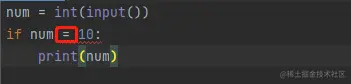correct ：### 8. Missing parameter self

Initialization function , Examples of function , Instance variables require default parameters self.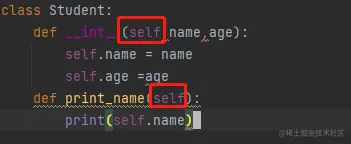### 9. Variable not defined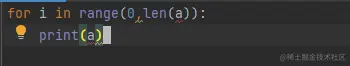Error message ：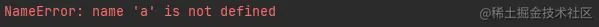Two 、 Code checklist

Here is a simple code checklist , Hope to be a little helpful for novices in programming , Only for reference , You can also summarize your programming mistakes .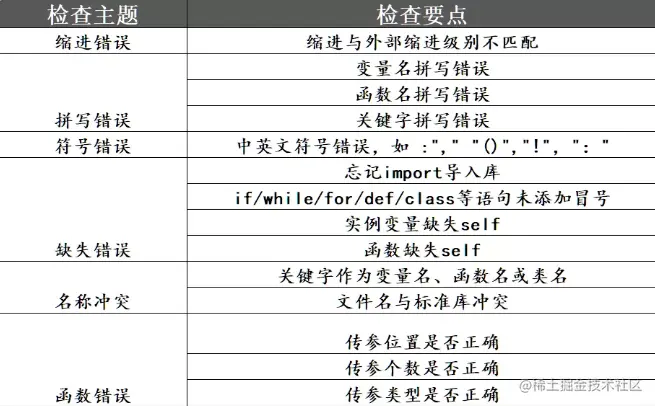3、 ... and 、 Novice entry applet

These examples are very simple and practical , Very suitable for beginners to practice . You can also try according to the purpose and tips of the project , Build your own solutions , Improve the level of programming .

1. Stone scissors and paper games

The goal is ： Create a command line game , Players can play in stone 、 Choose between scissors and cloth , With computers PK. If the player wins , The score will

add to , Until the end of the game , The final score will be shown to the player .

Tips ： Receive player's choice , And compared with the choice of the computer . The computer's selection is randomly selected from the selection list . If the player gets

- , Increase 1 branch .

``````import random
choices = ["Rock", "Paper", "Scissors"]
computer = random.choice(choices)
player = False
cpu_score = 0
player_score = 0
while True:
player = input("Rock, Paper or  Scissors?").capitalize()
#  Judge the choice of players and computers
if player == computer:
print("Tie!")
elif player == "Rock":
if computer == "Paper":
print("You lose!", computer, "covers", player)
cpu_score+=1
else:
print("You win!", player, "smashes", computer)
player_score+=1
elif player == "Paper":
if computer == "Scissors":
print("You lose!", computer, "cut", player)
cpu_score+=1
else:
print("You win!", player, "covers", computer)
player_score+=1
elif player == "Scissors":
if computer == "Rock":
print("You lose...", computer, "smashes", player)
cpu_score+=1
else:
print("You win!", player, "cut", computer)
player_score+=1
elif player=='E':
print("Final Scores:")
print(f"CPU:{cpu_score}")
print(f"Plaer:{player_score}")
break
else:
print("That's not a valid play. Check your spelling!")
computer = random.choice(choices)
Copy code ``````2. Send Email automatically

​ Purpose ： Write a Python Script , You can use this script to send email .

Tips ：email Libraries can be used to send e-mail .

``````import smtplib
from email.message import EmailMessage
email = EmailMessage()
email['from'] = 'xyz name'
email['to'] = 'xyz id'
email['subject'] = 'xyz subject'
email.set_content("Xyz content of email")
with smtlib.SMTP(host='smtp.gmail.com',port=587)as smtp:
## sending request to server
smtp.ehlo()
smtp.starttls()
smtp.send_message(email)
print("email send")
Copy code ``````

3.Hangman Little games

Purpose ： Create a simple command line hangman game .

Tips ： Create a list of password words and randomly select a word . Now underline each word “_” Express , Give users the chance to guess words , If the user guesses the right word , Will “_” Replace... With words .``````import time
import random
name = input("What is your name? ")
print ("Hello, " + name, "Time to play hangman!")
time.sleep(1)
print ("Start guessing...\n")
time.sleep(0.5)
## A List Of Secret Words
words = ['python','programming','treasure','creative','medium','horror']
word = random.choice(words)
guesses = ''
turns = 5
while turns > 0:
failed = 0
for char in word:
if char in guesses:
print (char,end="")
else:
print ("_",end=""),
failed += 1
if failed == 0:
print ("\nYou won")
break
guess = input("\nguess a character:")
guesses += guess
if guess not in word:
turns -= 1
print("\nWrong")
print("\nYou have", + turns, 'more guesses')
if turns == 0:
print ("\nYou Lose")
Copy code ``````

4. alarm clock

Purpose ： Write a program to create an alarm clock Python Script .

Tips ： You can use date-time Module to create alarm clock , as well as playsound The library plays sound .``````from datetime import datetime
from playsound import playsound
alarm_time = input("Enter the time of alarm to be set:HH:MM:SS\n")
alarm_hour=alarm_time[0:2]
alarm_minute=alarm_time[3:5]
alarm_seconds=alarm_time[6:8]
alarm_period = alarm_time[9:11].upper()
print("Setting up alarm..")
while True:
now = datetime.now()
current_hour = now.strftime("%I")
current_minute = now.strftime("%M")
current_seconds = now.strftime("%S")
current_period = now.strftime("%p")
if(alarm_period==current_period):
if(alarm_hour==current_hour):
if(alarm_minute==current_minute):
if(alarm_seconds==current_seconds):
print("Wake Up!")
break
Copy code ``````

5. Guess the number

Purpose ： Write a guess size Python Little games .

Tips ： You can use random Randomly generate numbers .

``````#  Guessing game man-machine battle
import random
target=random.randint(1,100)
count=0
while True:
guess=eval(input(' Please enter a guess integer （1 to 100）'))
count+=1
if guess>target:
print(' Guess the ')
elif guess<target:
print(' Guess a little ')
else:
print(' Guessed it ')
break
print(' The number of guesses in this round is :',count)
Copy code ``````6. Mathematical addition and subtraction

Purpose ： Write a mathematical calculation Python Little games .

Tips ： You can use random Randomly generated numbers add and subtract from each other , If you answer correctly, you can enter the next level .

``````from random import randint
level=0                    #0 Class start
while level<10:            # When the level is less than 10 Class time
a=randint(0,100)       # Randomly generate two digit integers
b=randint(0,100)
c=input(f"{a}+{b}=")
c=int(c)
if c==a+b:             # The calculation result is correct
level+=1           # Level plus 1
print(f" That's right ！ Now the level is {level} level , achieve 10 Class won ！")
else:                  # The calculation result is wrong
level-=1
print(f" Wrong answer ！ Now the level is {level}, achieve 10 Class won ！ Make persistent efforts ！")
print(f" Successfully achieve 10 level ！ Challenge success ！ awesome ！")
# Subtraction breakthrough games , Modify the above program , It should be noted that the two numbers should be compared before subtraction , Avoid negative numbers .
from random import randint
level=0                    #0 Class start
while level<10:            # When the level is less than 10 Class time
a=randint(0,100)       # Randomly generate two digit integers
b=randint(0,100)
if a>=b:
c=input(f"{a}-{b}=")
c=int(c)
if c==a+b:             # The calculation result is correct
level+=1           # Level plus 1
print(f" That's right ！ Now the level is {level} level , achieve 10 Class won ！")
else:                  # The calculation result is wrong
level-=1
print(f" Wrong answer ！ Now the level is {level}, achieve 10 Class won ！ Make persistent efforts ！")
print(f" Successfully achieve 10 level ！ Challenge success ！ awesome ！")
Copy code ``````

​7. Guess words

Purpose ： Write an English word to fill in Python Little games .

Tips ： You can use random Randomly generate an English word , If the answer is correct ！

``````import random

#  A list of words ( You can fill in the words you need to recite )
words = ["print", "int", "str", "len", "input", "format", "if","for","def"]

# Initialization information ↓↓↓↓↓↓↓
def init():
#  Declare three global variables
global word
global tips
global ranList

# Randomly pick up a word in the word list
word = list(words[random.randint(0, len(words) - 1)])

# List of random numbers , It holds random numbers that match the length of words （ No repetition ）
ranList = random.sample(range(0, len(word)), len(word))

# Store prompt information
tips = list()
# Initialization prompt message
# Store underscores that match the length of words
for i in range(len(word)):
tips.append("_")
# Two letters at random
tips[ranList] = word[ranList]
tips[ranList] = word[ranList]

# Function part ↓↓↓↓↓

# Display a menu
print(" Please enter '?'")
print(" To end the game, please enter 'quit!'")

# Display prompt message
def showtips():
for i in tips:
print(i, end=" ")
print()

# Need tips
def needTips(tipsSize):
# There are at least two unknown letters
if tipsSize <= len(word)-3:
tips[ranList[tipsSize]] = word[ranList[tipsSize]]
tipsSize += 1
return tipsSize
else:
print(" There is no hint ！")

# Main running function ↓↓↓↓↓↓
def run():
print("------python Keyword version -------")
init()
tipsSize = 2

while True:
print(" Tips ：",end="")
showtips()
guessWord = input(" Guess the word ：")
# ''.join(word)> hold word The contents of the list are converted to strings
if guessWord == ''.join(word):
print(" congratulations , Guessed it ！ Namely %s!"%(''.join(word)))
print(" Guess again ")
init()

elif guessWord == '?':
tipsSize = needTips(tipsSize)
elif guessWord == 'quit!':
break
else:
print(" Wrong guess. ！")
continue
run()
Copy code ``````8. Face detection

Purpose ： Write a Python Script , You can detect faces in images , And save all the faces in one folder .

Tips ： have access to haar Cascade classifiers detect faces . It returns face coordinate information , It can be saved in a file .

``````import cv2
# Read the input image
# Convert into grayscale
gray = cv2.cvtColor(img, cv2.COLOR_BGR2GRAY)
# Detect faces
faces = face_cascade.detectMultiScale(gray, 1.3, 4)
# Draw rectangle around the faces
for (x, y, w, h) in faces:
cv2.rectangle(img, (x, y), (x+w, y+h), (255, 0, 0), 2)
crop_face = img[y:y + h, x:x + w]
cv2.imwrite(str(w) + str(h) + '_faces.jpg', crop_face)
# Display the output
cv2.imshow('img', img)
cv2.imshow("imgcropped",crop_face)
cv2.waitKey()
Copy code ``````

9. Keyboard recorder

​ Purpose ： Write a Python Script , Save all the keys pressed by the user in a text file .

Tips ：pynput yes Python One of the Libraries , It is used to control the movement of keyboard and mouse , It can also be used to make keyboard recorders . Simply read the user press

The key , And save them in a text file after a certain number of keys .

``````from pynput.keyboard import Key, Controller,Listener
import time
keyboard = Controller()

keys=[]
def on_press(key):
global keys
#keys.append(str(key).replace("'",""))
string = str(key).replace("'","")
keys.append(string)
main_string = "".join(keys)
print(main_string)
if len(main_string)>15:
with open('keys.txt', 'a') as f:
f.write(main_string)
keys= []
def on_release(key):
if key == Key.esc:
return False

with listener(on_press=on_press,on_release=on_release) as listener:
listener.join()
Copy code ``````

10. Short URL generatorPurpose ： Write a Python Script , Use API Shorten a given URL.

``````from __future__ import with_statement
import contextlib
try:
from urllib.parse import urlencode
except ImportError:
from urllib import urlencode
try:
from urllib.request import urlopen
except ImportError:
from urllib2 import urlopen
import sys

def make_tiny(url):
request_url = ('http://tinyurl.com/api-create.php?' +
urlencode({'url':url}))
with contextlib.closing(urlopen(request_url)) as response:

def main():
for tinyurl in map(make_tiny, sys.argv[1:]):
print(tinyurl)

if __name__ == '__main__':
main()
-----------------------------OUTPUT------------------------
python url_shortener.py https://www.wikipedia.org/
https://tinyurl.com/buf3qt3
Copy code ``````

## summary

All right. ！ That's what I want to share with you today , If necessary, you can collect it and look at it slowly ~

While watching, you should also try to type the code yourself ！

### Complete free source code collection office ：

For complete project source code + See... For the source code base of the material ：# Private letter editor 06# Or click the blue text to add to get free benefits ！

Your support is my biggest motivation ！！ Remember Sanlian ~mua Welcome to read previous articles ~

### New answers ——

If you are learning Python Problems encountered in 、 I'll give you an answer when I have time ！ You need to add it yourself ！

discharge WX The number is as follows ：apython68

## A summary of the article ——

Python—2021 | Summary of existing articles | Continuous updating , Just read this article directly ~